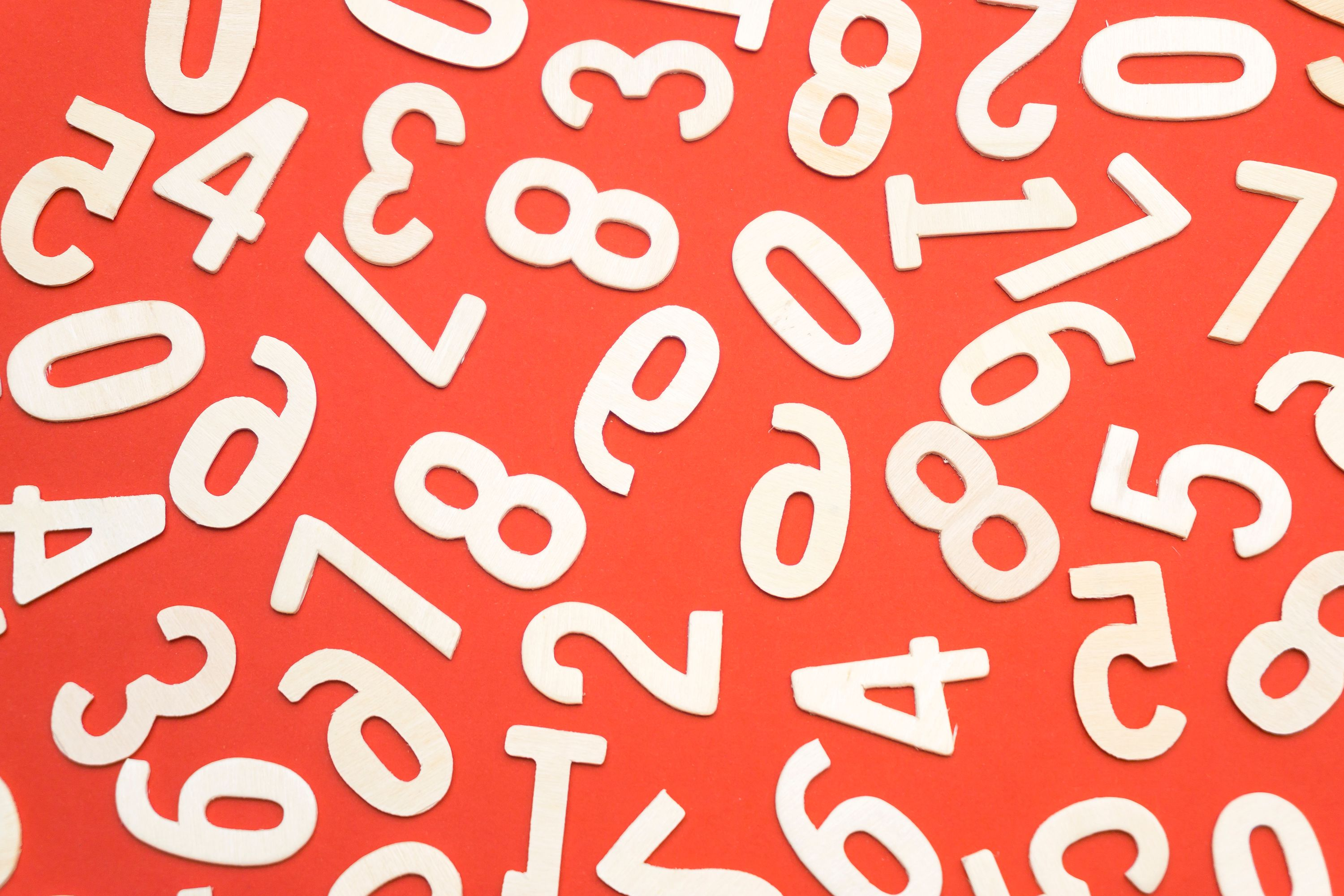## Arbitrary Precision Integers – creating from normal integers

Some months ago I was in the need for an arbitrary precision integer class for one of my Haxe games. Although the Haxe library is quite exhaustive, there was no such class. So I decided to write my own version, and I learned a lot from that. You can find it on github and in the haxe library system.

They are put to action in the clicker-style game Idle Snowflake And now I want to share all my insights with you.This tutorial will handle some math stuff. So you should know how basic operators work and you should be familiar with the basics of binary and decimal number representations.

Although my library was written in Haxe, the algorithms and concepts are universal and can be applied in any language.

## Requirements

The requirements for my arbitrary precision integers (API) are:

• APIs can be created from normal integers
• APIs can create their string representation in binary as well as decimal
• An API can be converterd to a normal integer if the size is fitting
• the usual mathematical operations are possible (+, -, *, /, pow, modulo, left shift, right shift, compare, calc logarithm)
• Fully tested interface

Negative numbers are not required. I had no special requirements regarding memory or performance. My APIs are written in a way that you can create them as large as possible. However, they might become slow and at some point you might run out of system memory. Optimizations for special use cases are possible, however not required.

## Basic representation:

I decided to go for a binary representation of numbers. This was mainly due to the fact that I really like binary numbers. So the basic building block of the API is just

``public var values: Array<Bool> = [];``

Which is just an array of booleans.

## Craete an API from a normal integer

Ok, so lets dig into the first problem: How to create an API from a normal integer. At first we have to check whether the integer we want to create an API from is negative. Because we don't deal with that, I just throw an error in that case.

If the passed integer is zero, than we just return a binary representation which has exactly one `false` value in the array.

As those easy cases are fixed, we now can tackle the actual work to be done. This will be done in a loop as long as we have treated the complete number. The first thing to do is to check for the end condition, which is just checking if the current value is zero. Then we just break the loop.

If this is not the case, each loop iteration will check for the reminder (modulo) when the number is divided by two. The result can either be zero or one. Thus we either push `false` or `true` into the array. After that, the number is rightshifted by one (division by two). This happens until we reached the final representation of two and the number is zero, which will subsequentially exit the loop. As we were pushing to the array from the left, the order of the array has to be reversed to ensure the correct endianess. With this part of the code, we can convert numbers in binary representation. The following list gives an example of some numbers, decimal on the left, binary on the right:

``````4   =     100
10  =    1010
42  =  101010
100 = 1100100
101 = 1100101``````

As we can only feed normal integers there is no arbitrary presicion yet, but that will come once we have addition, pow and multiplication, which will be covered in another tutorial.